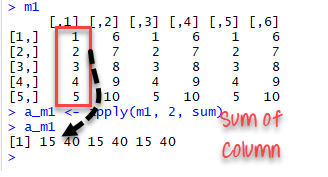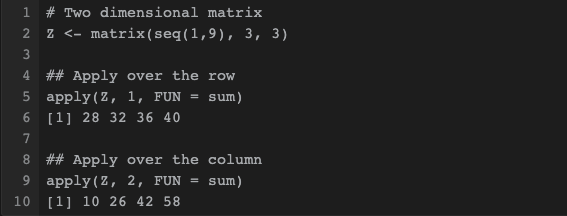# What is apply() function?

apply() takes Data frame or matrix as an input and gives output in vector, list or array. Apply function in R is primarily used to avoid explicit uses of loop constructs. It is the most basic of all collections can be used over a matrice.

This function takes 3 arguments:

apply(X, MARGIN, FUN) Here: -x: an array or matrix -MARGIN: take a value or range between 1 and 2 to define where to apply the function: -MARGIN=1`: the manipulation is performed on rows -MARGIN=2`: the manipulation is performed on columns -MARGIN=c(1,2)` the manipulation is performed on rows and columns -FUN: tells which function to apply. Built functions like mean, median, sum, min, max and even user-defined functions can be applied>

The simplest example is to sum a matrice over all the columns. The code apply(m1, 2, sum) will apply the sum function to the matrix 5x6 and return the sum of each column accessible in the dataset.

m1 ← matrix(C<-(1:10),nrow=5, ncol=6) m1 a_m1 ← apply(m1, 2, sum) a_m1

Output:apply() function example in R

Best practice: Store the values before printing it to the console.

The apply() family pertains to the R base package and is populated with functions to manipulate slices of data from matrices, arrays, lists and dataframes in a repetitive way. These functions allow crossing the data in a number of ways and avoid explicit use of loop constructs. They act on an input list, matrix or array and apply a named function with one or several optional arguments.